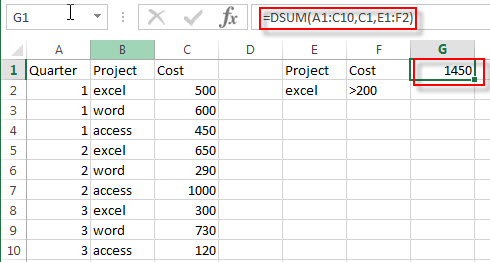## Excel DSUM Function

This post will guide you how to use Excel DSUM function with syntax and examples in Microsoft excel.

### Description

The Excel DSUM Function will add the numbers in a column or database that meets a given criteria. And so you can use the DSUM function to add the numbers in a field of records in a list or database that match a given conditions that you specify in Excel.

The DSUM function is a build-in function in Microsoft Excel and it is categorized as a Database Function.

The DSUM function is available in Excel 2016, Excel 2013, Excel 2010, Excel 2007, Excel 2003, Excel XP, Excel 2000, Excel 2011 for Mac.

### Syntax

The syntax of the DSUM function is as below:

`= DSUM (database, field, criteria)`

Where the DSUM function arguments are:

• Database -This is a required argument. The range of cells that containing the database.
• Field – This is a required argument. The column with database that you want the minimum of.
• Criteria – The range of cells that contains the conditions that you specify.

### Excel DSUM Function Examples

The below examples will show you how to use Excel DSUM Function to get the sum of values from a set of records that match a given criteria.

#1 to get the total cost from excel project and its cost is greater than 200, using the following formula:

`=DSUM(A1:C10,C1,E1:F2)`